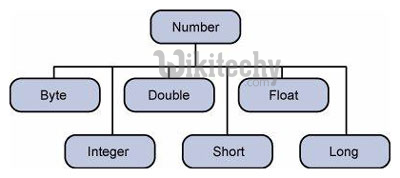# java tutorial - Java Numbers - java programming - learn java- java basics - java for beginnersLearn Java - Java tutorial - Java number - Java examples - Javaprograms

Usually, when working with numbers in Java, we use primitive data types, such as byte, int, long, double, etc.

## Sample Code

• ТHowever, in development, we encounter situations where we need to use objects instead of primitive data types. In order to achieve this, Java provides wrapper classes.
• All shell classes (Integer, Long, Byte, Double, Float, Short) are subclasses of the abstract class of numbers in Java (class Number).Learn java - java tutorial - java number - java examples - java programs

• The wrapper object contains or wraps its corresponding primitive data type. Converting primitive data types to an object is called wrapping, and the compiler takes care of this.
• Therefore, when using a wrapper class, you simply pass the value of the primitive data type to the constructor of the wrapper class.
• The shell object can be converted back to a primitive data type, and this process is called unpacking.
• The number class is part of the java.lang package.

Here is an example of packaging and unpacking:

## Output

• When x is assigned an integer value, the compiler packs an integer, because x is an integer object. Later, it decompresses x so that it can be added as an integer.

## Methods of the class of numbers

NO Methods Description
1 xxxValue() It changes the value of an integer object to a xxx data type and returns it.
2 compareTo() Relates an integer object with an argument.
3 equals() Specifies whether the integer is an argument.
4 valueOf() Returns an integer object holding the specified value.
5 toString() Returns a string object (String) that represents the specified int value or an integer object.
6 parseInt() The method is used to take a primitive data type of a specific string.
7 abs() Returns the absolute value of the argument.
8 ceil() It returns lowest double value which is greater than or equal to argument then equivalent to a accurate integer.
9 floor() It returns biggest double value which is less than or equal to argument then equivalent to a accurate integer .
10 rint() The method rint returns the integer that is closest in value to the argument.
11 round() Returns the nearest long or int to the argument according to the rounding rules.
12 min() Returns the smaller of the two arguments.
13 max() Returns the larger of the two arguments.
14 exp() Returns the number e of Euler, raised to the power of a double value.
15 log() Returns the natural logarithm (based on e) with a double value.
16 pow() Returns the value of the initial argument raised to the power of the next argument.
17 sqrt() Returns a suitable rounded positive square root of a double value
18 sin() Returns the sine of the identified double value.
19 cos() Returns the cosine of the identified double value.
20 tan() Returns the tangent of the identified double value.
21 asin() Returns the arcsine of the identified double value.
22 acos() Returns the arc cosine of the identified double value.
23 atan() Returns the arc tangent of the identified double value.
24 atan2() Returns the theta angle from the transformation of rectangular coordinates (x, y) in polar coordinates (g, theta).
25 toDegrees() Converts an angle measured in radians to approximately the equivalent angle measured in degrees.
26 toRadians() Converts the angle measured in degrees to approximately the equivalent angle measured in radians.
27 random() Returns a double value with a positive sign, greater than or equal to 0.0, too less than 1.0.